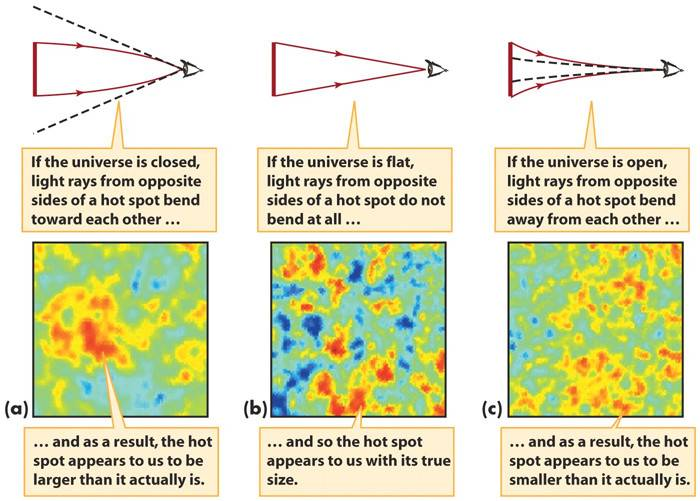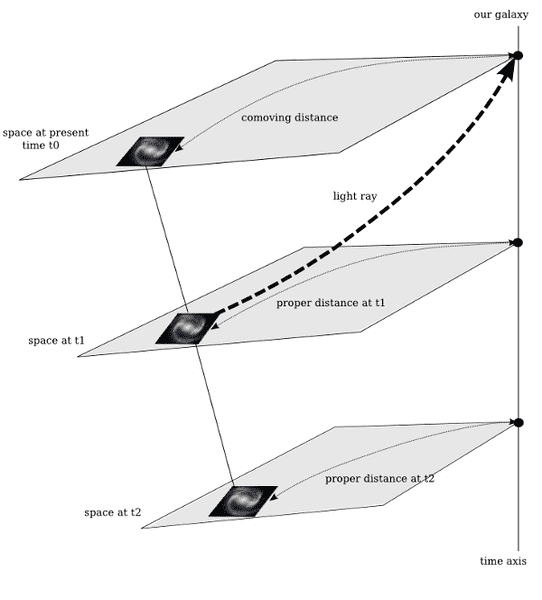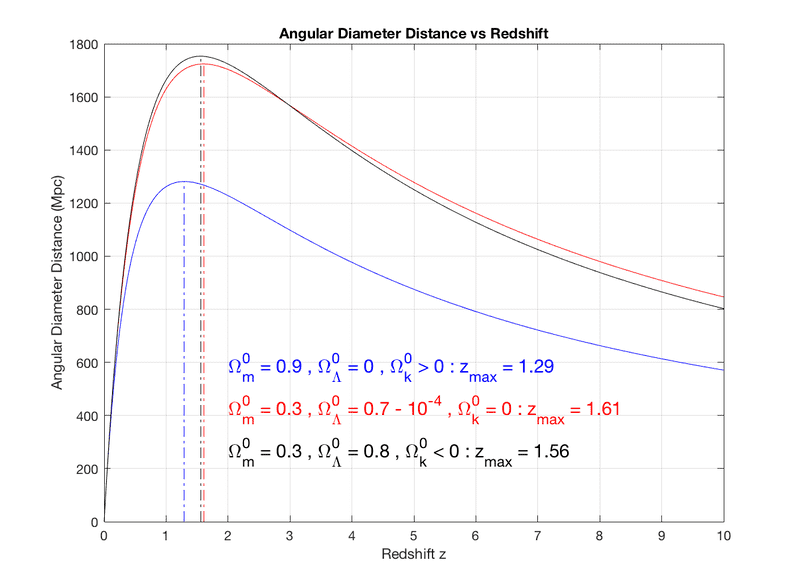# Trying to reproduce curves with angle of CMB anisotropies

• I
I am looking for a way to get, by a simple numerical computation, the 3 curves on the following figure:For this, I don't know what considering as abcissa (comoving distance ?, i.e

##D_{comoving} = R(t)r##

with ##R(t)## scale factor and ##r## the coordinate which appears into FLRW metric).

Previously, I found a little project about the trajectory of light geodesics in an expanding universe; here below a figure illustrating the expected results with FLRW metric:As you can see, the curve of light is bended since $\Lambda\text{CDM}$ current model produces acceleration of expansion, so light has more and more difficulties to reach our galaxy.

In a first version of this project, I have computed a light geodesic in Einstein-de-Sitter universe : so in this case, the distance between the 2 galaxies (emitting and our galaxy) which is equal to :

##D_{{\varphi}}=\text{Distance}_{init}\,\bigg[\frac{3\,H_{0}}{2c}\,ct\bigg]^{2/3}##

I did also a simple computation on the angular diameter distance versus the redshift. I get this figure which is the expected results for the 3 models (k=-1,0,1) :I would have thought that angle of anistropies was constant during its travel : that is not the case on the first figures at the top of post, which illustrates a bending, producing then a different angle between the initial emission and the final reception by our eyes : **is it actually right ?**

I think my main issue, to produce this first figure, is to know what I have got to take as abscissa ? the comoving distance, the proper distance, the angular diameter distance ?

Firstly, I believed that I should take the comoving distance but I don't know how to make converge 2 light rays (extremities of one anisotropy) in oberver eyes.

Any help is welcome to reproduce the 3 curves on the first figure of this post : these curves illustrate very well the notion of smaller/bigger value of apparent anisotropies as a function of curvature parameter.